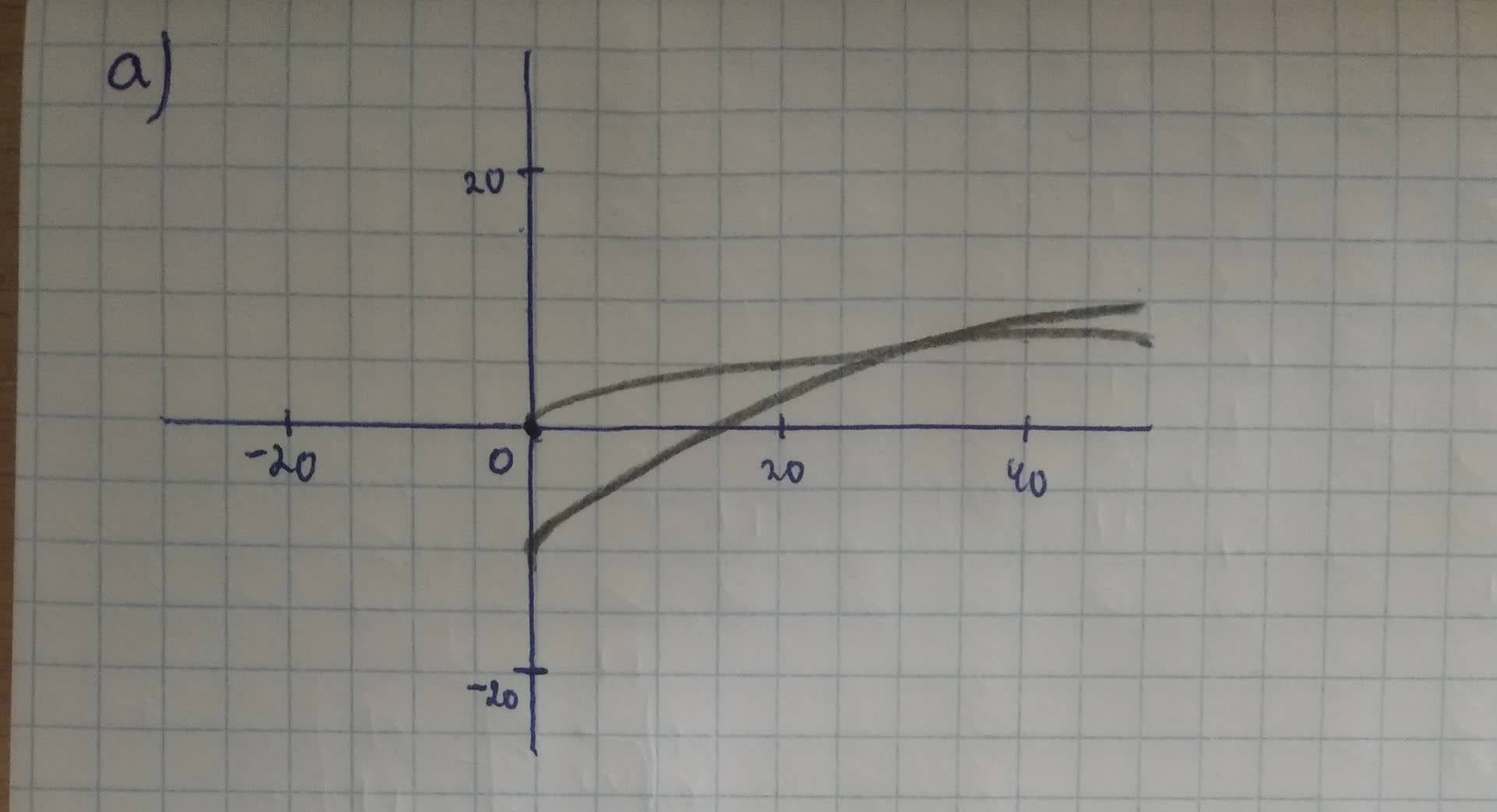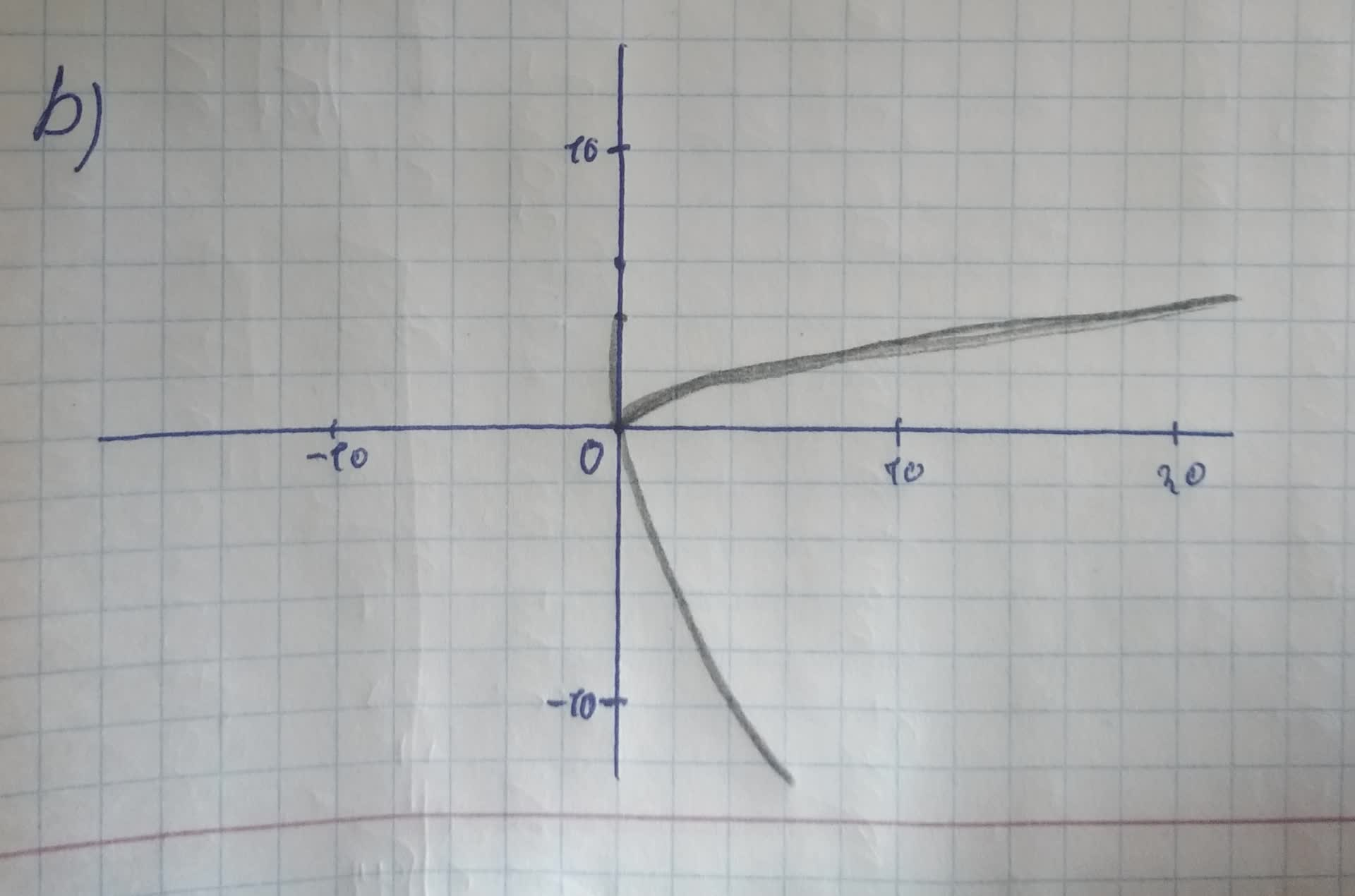Question# What transformations of the parent graph of f(x) = \sqrtc

Transformations of functions
ANSWEREDWhat transformations of the parent graph of $$\displaystyle{f{{\left({x}\right)}}}=\sqrt{{c}}$$ produce the graphs of the following functions?
a) $$\displaystyle{m}{\left({x}\right)}=\sqrt{{{7}{x}-{3.5}}}-{10}$$
b) $$\displaystyle{j}{\left({x}\right)}=-{2}\sqrt{{{12}{x}}}+{4}$$2021-08-15

a)First, we have to rewrite the function m(x) in the form $$\displaystyle{m}{\left({x}\right)}=\sqrt{{{x}—{h}}}+{k}$$
So, the steps are: factor the radicand, use the Product Property of Radicals and than simplify, so, we have next:
$$\displaystyle{m}{\left({x}\right)}=\sqrt{{{7}{\left({x}-{0.5}\right)}}}-{10}$$
$$\displaystyle=\sqrt{{7}}\cdot\sqrt{{{x}-{0.5}}}-{10}$$
$$\displaystyle={2.65}\sqrt{{x}}={0.5}-{10}$$
So, the graph of m(x) is a vertical stretch of a parent function by a factor of 2.65 or $$\displaystyle\sqrt{{7}}$$, translated right 0.5 units and translated down 10 units. On the following picture there is a graph of $$\displaystyle{y}=\sqrt{{x}}{\quad\text{and}\quad}{m}{\left({x}\right)}=\sqrt{{{7}{x}-{3.5}}}-{10}$$b)First, we have to rewrite the function j(x) in the form: $$\displaystyle{j}{\left({x}\right)}={a}\sqrt{{{x}-{h}}}+{k}$$ So, the steps are: use the Product Property of Radicals and than simplify, so we have next:
$$\displaystyle{j}{\left({x}\right)}=-{2}\sqrt{{{4}\cdot{3}\cdot{x}}}+{4}$$
$$\displaystyle=-{2}\sqrt{{4}}\cdot\sqrt{{3}}\cdot\sqrt{{x}}+{4}$$
$$\displaystyle=-{2}\cdot{2}\cdot\sqrt{{3}}\cdot\sqrt{{x}}+{4}$$
$$\displaystyle=-{4}\sqrt{{3}}\cdot\sqrt{{x}}+{4}$$
So, the graph of j(x) is a vertical stretch of a parent function by a factor of $$\displaystyle-{4}\sqrt{{3}}$$, translated up 4 units.